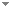Switch to: References

# Programming Planck units from a virtual electron; a Simulation Hypothesis

 Programming Planck Units From a Virtual Electron; a Simulation Hypothesis (Summary).Malcolm Macleod - 2018 - Eur. Phys. J. Plus 133:278.The Simulation Hypothesis proposes that all of reality, including the earth and the universe, is in fact an artificial simulation, analogous to a computer simulation, and as such our reality is an illusion. In this essay I describe a method for programming mass, length, time and charge (MLTA) as geometrical objects derived from the formula for a virtual electron; $f_e = 4\pi^2r^3$ ($r = 2^6 3 \pi^2 \alpha \Omega^5$) where the fine structure constant $\alpha$ = 137.03599... and $\Omega$ = 2.00713494... (...) Direct download (2 more)     Export citationBookmarkOutlined here is a simulation hypothesis approach that uses an expanding (the simulation clock-rate measured in units of Planck time) 4-axis hyper-sphere and mathematical particles that oscillate between an electric wave-state and a mass (unit of Planck mass per unit of Planck time) point-state. Particles are assigned a spin axis which determines the direction in which they are pulled by this (hyper-sphere pilot wave) expansion, thus all particles travel at, and only at, the velocity of expansion (the origin of $c$), (...) Direct download (2 more)     Export citationBookmarkThe Simulation Hypothesis proposes that all of reality is in fact an artificial simulation, analogous to a computer simulation. Outlined here is a method for programming relativistic mass, space and time at the Planck level as applicable for use in Planck Universe-as-a-Simulation Hypothesis. For the virtual universe the model uses a 4-axis hyper-sphere that expands in incremental steps (the simulation clock-rate). Virtual particles that oscillate between an electric wave-state and a mass point-state are mapped within this hyper-sphere, the oscillation driven (...) Direct download (2 more)     Export citationBookmarkDefined are gravitational formulas in terms of Planck units and units of $\hbar c$. Mass is not assigned as a constant property but is instead treated as a discrete event defined by units of Planck mass with gravity as an interaction between these units, the gravitational orbit as the sum of these mass-mass interactions and the gravitational coupling constant as a measure of the frequency of these interactions and not the magnitude of the gravitational force itself. Each particle that is (...) Direct download (2 more)     Export citationBookmarkFollowing the 26th General Conference on Weights and Measures are fixed the numerical values of the 4 physical constants ($h, c, e, k_B$). This is premised on the independence of these constants. This article discusses a model of a mathematical electron from which can be defined the Planck units as geometrical objects (mass M=1, time T=2$\pi$ ...). In this model these objects are interrelated via this electron geometry such that once we have assigned values to 2 Planck units then we (...) Direct download     Export citationBookmark